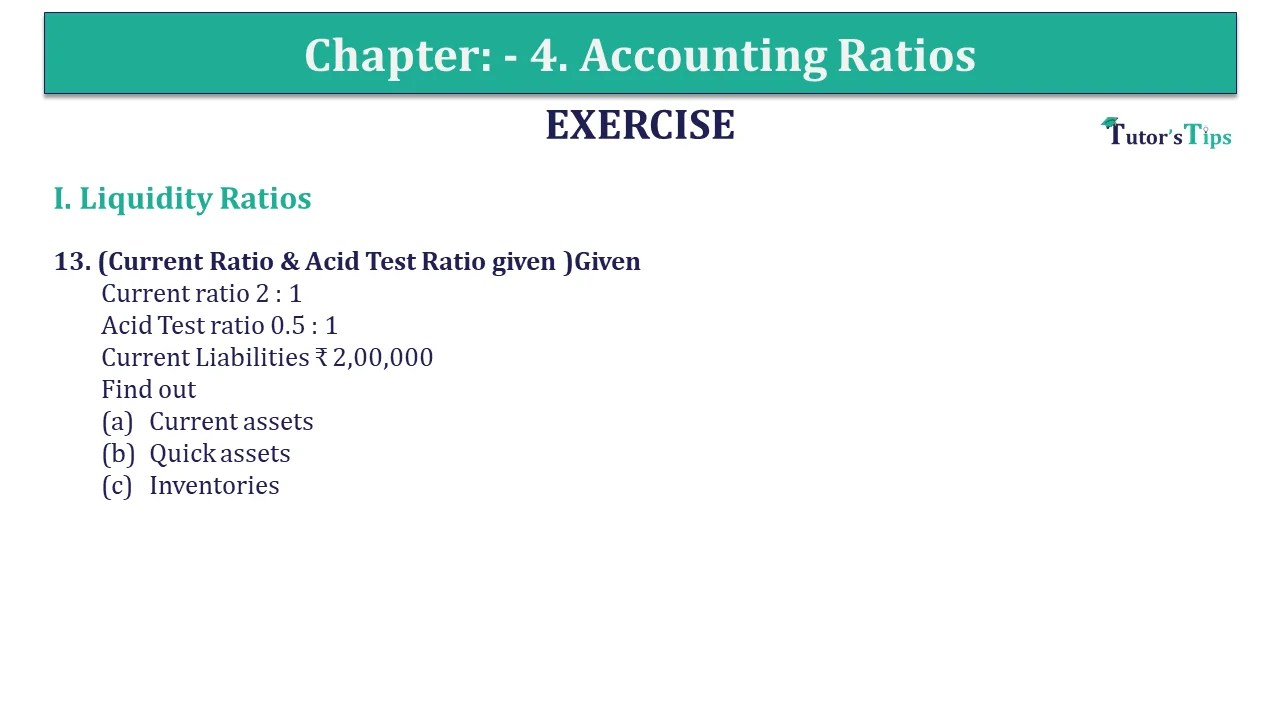# Question 13 Chapter 4 of +2-B – USHA Publication 12 ClassQuestion 13 Chapter 4 of +2-B

I. Liquidity Ratios

13. (Current Ratio & Acid Test Ratio given )Given
The current ratio of 2: 1
Acid Test ratio of 0.5: 1
Current Liabilities ₹ 2,00,000
Find out
Current assets
Quick assets
Inventories

## The solution of Question 13 Chapter 4 of +2-B: –

Solution: – (a)

 Current Ratio = 2 : 1 Current Liabilities = 2,00,000 Current Assets = Current Liabilities x 2 = ₹ 2,00,000 x 2 = ₹ 4,00,000

Solution: – (b)

 Quick Ratio = 0.5 : 1 Current Liabilities = ₹ 2,00,000 Quick Assets = Current Liabilities x 0.5 = ₹ 2,00,000 x 0.5 = ₹ 1,00,000

Solution: – (c)

 Inventories = Current Assets – Quick Assets = ₹ 4,00,000 – ₹ 1,00,000 = ₹ 3,00,000

What are Liquidity Ratios – Formulas and Examples

Comment if you have any question.

Also, Check out the solved question of previous Chapters: –